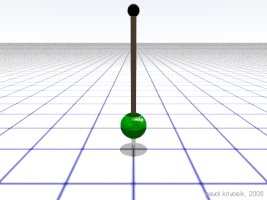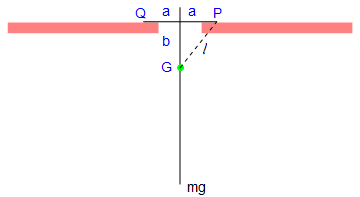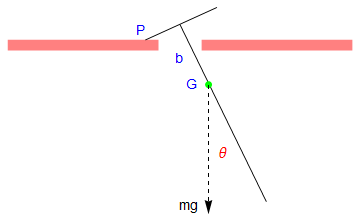# Preface

This section discusses differential equation used to model pendulum vibrations.

# Pendulum

There are many examples in real life when an object rotates around a particular center. Everyone knows that the planets orbit the Sun in a circular orbit, right? Well ... not exactly. A 17th century mathematician by the name of Johannes Kepler was able to show that the orbits of planets about the sun are elliptical in shape.

For a circular motion, it is convenient to use polar coordinates (r,θ), where r is the distance from the center of rotation and θ is the angle from the reference line, which is usually identified with a positive semi-axis of ordinate in rectangular coordinates. For motion in a circle of radius r, the circumference of the circle is C = 2π r. If the period for one rotation is T, the angular rate of rotation, also known as angular velocity, ω is:

$\omega = \frac{2\pi}{T} = 2\pi \nu = \frac{{\text d}\theta}{{\text d}t} \qquad \mbox{with units radians/second or simply 1/second}.$
The speed of the object traveling the circle is:
$v = \frac{2\pi r}{T} = \omega r .$
The angle θ swept out in a time t is:
$\theta = 2\pi \,\frac{t}{T} = \omega t .$
The angular acceleration, α, of the particle is:
$\alpha = \frac{{\text d}\omega}{{\text d}t} = \frac{{\text d}^2 \theta}{{\text d}t^2} .$
In the case of uniform circular motion, α will be zero. The angular acceleration of a point particle α can be connected to the applied torque τ by the following equation:
$I \alpha = {\bf \tau} - 2mr\,\frac{{\text d}r}{{\text d}t} \,\omega ,$
where m is the mass of the particle and I is its moment of inertia.

The SI unit for torque is N⋅m. The symbol for torque is typically τ, the lowercase Greek letter τ. In mechanical engineering, it is called moment of force, and is commonly denoted by M. The term torque was introduced into English scientific literature by James Thomson, the brother of Lord Kelvin, in 1884. When force F is applied to a circular rotated object, τ = r × F (with × denoting the cross product). Alternatively,

$\tau = \| {\bf r} \| \cdot \| {\bf F} \| \,\sin \theta = r\,F\,\sin \theta = r\,F_{\perp} ,$
where F is the amount of force directed perpendicularly to the position of the particle. The unbalanced torque on a body along axis of rotation determines the rate of change of the body's angular momentum,
${\bf \tau} = \frac{{\text d}{\bf L}}{{\text d}t} ,$
where L is the angular momentum vector and t is time. The definition of angular momentum for a single particle is: L = r × p, where "×" indicates the vector cross product, p is the particle's linear momentum, and r is the displacement vector from the origin (the origin is assumed to be a fixed location anywhere in space).

Taking the derivative, we obtain

$\frac{{\text d}{\bf L}}{{\text d}t} = {\bf r} \times \frac{{\text d}{\bf p}}{{\text d}t} + \frac{{\text d}{\bf r}}{{\text d}t} \times {\bf p} = {\bf r} \times \frac{{\text d}{\bf p}}{{\text d}t} = {\bf r} \times {\bf F}$
because the cross product or the derivative of r and p is zero (these two vectors are parallel). Therefore, torque on a particle is equal to the first derivative of its angular momentum with respect to time.

The acceleration due to change in the direction is:

$a = \frac{v^2}{r} = \omega^2 r .$
The centripetal force
$F_c = \frac{m\, v^2}{r} ,$
and centrifugal force can also be found out using acceleration:
${\bf F} = m \left[ \frac{{\text d}^2 {\bf r}}{{\text d} t^2} \right] .$
The simple pendulum is of historic and basic importance. Its approximate isochronism was first discovered by Galileo, who is said to check the constancy of the period of the small oscillations of a pendulum by comparing them with his heartbeat. In hands of Newton, pendulum provided the first evidence that inertial and gravitational masses are proportional. Until relatively recently, the plane pendulum yielded the most accurate and convenient method for measuring the local gravitational acceleration.

There is no doubt that the first person who investigated and established the mathematical theory and properties of the pendulum was a prominent Dutch mathematician and scientist named Christiaan Huygens (this spelling of his name is taken from the title of his 1658 book Horologium Oscillatorium). The main point of Huygens' (1629--1695) discovery was that the curve in which a pendulum particle (or bob) hangs by a string of insensible weight must move in order to be isochronous in its vibrations, and is not a circle, but a cycloid.

The pendulum consists of a bob (point mass) of mass m attached to the end of a light inextensible rod of length ℓ and negligible weight, with the motion taking place in a vertical plane. Such pendulum is usually referred to as a simple pendulum. Let θ be the angular coordinate of m measured counterclockwise from the down position. We model it as a particle constrained to move in a circle of radius ℓ. Upon choosing rectangular coordinates with the origin at the pivot point and assuming that the pendulum oscillates within the plane, then we label the vertical axis with y so that the pendulum moves in the plane of the circle having the center being the origin. Because the mass must move on the circle, at each time t we have

$x^2 (t) + y^2 (t) = \ell^2 , \qquad z(t) \equiv 0 ,$
and we can use an angle θ to characterize the configuration of the pendulum; as usual,
$x = \ell \,\sin \theta , \qquad y = -\ell + \ell\, \cos \theta .$## IV. Driven Pendulum

A driven pendulum may exhibit a chaotic motion. It consists of a mass m fixed at a distance $$\ell$$ from a pivot which is subject to a vertical oscillation $$y = A\, \cos \left( \omega \, t \right) .$$ Let θ be the angular coordinate of m measured counterclockwise from the down position, and $$\phi = \pi - \theta$$ the complementary displacement measured clockwise from the up orientation. The kinetic and potential energies are

## V. Rocking Rigid Pendulum

plus = Polygon[{{-1/2, 0}, {-1/2, 1/4}, {-4, 1/4}, {-4, 0}}]
minus = Polygon[{{1/2, 0}, {1/2, 1/4}, {4, 1/4}, {4, 0}}]
a = Show[Graphics[{Pink, minus}], Graphics[{Pink, plus}]]
line1 = Graphics[Line[{{-2, -3.5}, {0, 0.6}}], PlotRange -> {{-2, 2}, {-4, 1}}]
line2 = Graphics[Line[{{-0.85, 1.0}, {0.8, 0.25}}], PlotRange -> {{-2, 2}, {-4, 1}}]
makeArrowPlot[g_Graphics, ah_: 0.05, dx_: 1*^-6, dy_: 1*^-6] :=
Module[{pr = PlotRange /. Options[g, PlotRange], gg, lhs, rhs},
gg = g /. GraphicsComplex -> (Normal[GraphicsComplex[##]] &);
lhs := Or @@
Flatten[{Thread[Abs[#[[1, 1, 1]] - pr[]] < dx], Thread[Abs[#[[1, 1, 2]] - pr[]] < dy]}] &;
rhs := Or @@ Flatten[{Thread[Abs[#[[1, -1, 1]] - pr[]] < dx], Thread[Abs[#[[1, -1, 2]] - pr[]] < dy]}] &;
gg = gg /.
x_Line?(lhs[#] && rhs[#] &) :> {Arrowheads[{-ah, ah}], Arrow @@ x};
gg = gg /. x_Line?lhs :> {Arrowheads[{-ah, 0}], Arrow @@ x};
gg = gg /. x_Line?rhs :> {Arrowheads[{0, ah}], Arrow @@ x};
gg]
curve = Plot[{-Sqrt[9 - x^2]}, {x, -2.2, 2.2}, PlotStyle -> {Thick, Dashed}, Axes -> False] // makeArrowPlot
Show[a, line1, line2, curve]

A rigid pendulum, such as a long rod, has a short cross-bar rigidly attached to it. As it oscillates in vertical plane, it is supported on ends of the cross-bar as they alternately contact a fixed horizontal surface:
plus = Polygon[{{-1/2, 0}, {-1/2, 1/4}, {-4, 1/4}, {-4, 0}}]
minus = Polygon[{{1/2, 0}, {1/2, 1/4}, {4, 1/4}, {4, 0}}]
a = Show[Graphics[{Pink, minus}], Graphics[{Pink, plus}]]
line1 = Graphics[Line[{{-2, -3.5}, {0, 0.6}}], PlotRange -> {{-2, 2}, {-4, 1}}]
line2 = Graphics[Line[{{-0.85, 1.0}, {0.8, 0.25}}], PlotRange -> {{-2, 2}, {-4, 1}}]
p2 = Graphics[{Dashed, Arrow[{{-0.66, -0.8}, {-0.66, -3.8}}]}]
point = Graphics[{PointSize[Large], Green, Point[{-0.66, -0.8
t1 = Graphics[ Text[Style["$Theta]", FontSize -> 14, Red], {-1.0, -2.4}]] t2 = Graphics[Text[Style["G", FontSize -> 14, Blue], {-1.0, -0.8}]] a1 = Graphics[Text[Style["a", FontSize -> 14, Blue], {0.5, 0.7}]] a2 = Graphics[Text[Style["a", FontSize -> 14, Blue], {-0.2, 1.0}]] Q = Graphics[Text[Style["Q", FontSize -> 14, Blue], {1.0, 0.45}]] b = Graphics[Text[Style["b", FontSize -> 14, Blue], {0.0, -0.2}]] mg = Graphics[Text[Style["mg", FontSize -> 14, Black], {-0.2, -3.6}]] Show[a, line1, line2, point, p2, t1, t2, a1, a2, Q, b, mg] plus = Polygon[{{-1/2, 0}, {-1/2, 1/4}, {-4, 1/4}, {-4, 0}}] minus = Polygon[{{1/2, 0}, {1/2, 1/4}, {4, 1/4}, {4, 0}}] a = Show[Graphics[{Pink, minus}], Graphics[{Pink, plus}]] line1 = Graphics[Line[{{2, -3.5}, {0, 0.6}}], PlotRange -> {{-2, 2}, {-4, 1}}] line2 = Graphics[Line[{{0.85, 1.0}, {-0.8, 0.25}}], PlotRange -> {{-2, 2}, {-4, 1}}] p2 = Graphics[{Dashed, Arrow[{{0.66, -0.8}, {0.66, -3.8}}]}] point = Graphics[{PointSize[Large], Green, Point[{0.66, -0.8}]}] t1 = Graphics[ Text[Style["\[Theta]", FontSize -> 14, Red], {1.0, -2.4}]] t2 = Graphics[Text[Style["G", FontSize -> 14, Blue], {0.3, -0.8}]] P = Graphics[Text[Style["P", FontSize -> 14, Blue], {-0.9, 0.45}]] b = Graphics[Text[Style["b", FontSize -> 14, Blue], {0.0, -0.2}]] mg = Graphics[Text[Style["mg", FontSize -> 14, Black], {0.22, -3.6}]] Show[a, line1, line2, point, p2, t1, t2, P, b, mg] plus = Polygon[{{-1/2, 0}, {-1/2, 1/4}, {-4, 1/4}, {-4, 0}}] minus = Polygon[{{1/2, 0}, {1/2, 1/4}, {4, 1/4}, {4, 0}}] a = Show[Graphics[{Pink, minus}], Graphics[{Pink, plus}]] line1 = Graphics[Line[{{0, -3.5}, {0, 0.6}}], PlotRange -> {{-2, 2}, {-4, 1}}] line2 = Graphics[Line[{{0.85, 0.27}, {-0.85, 0.27}}], PlotRange -> {{-2, 2}, {-4, 1}}] P = Graphics[Text[Style["P", FontSize -> 14, Blue], {0.9, 0.45}]] Q = Graphics[Text[Style["Q", FontSize -> 14, Blue], {-0.9, 0.45}]] mg = Graphics[Text[Style["mg", FontSize -> 14, Black], {0.4, -3.6}]] point = Graphics[{PointSize[Large], Green, Point[{0, -0.8}]}] t2 = Graphics[Text[Style["G", FontSize -> 14, Blue], {-0.3, -0.8}]] p3 = Graphics[{Dashed, Line[{{0, -0.8}, {0.85, 0.27}}]}] b = Graphics[Text[Style["b", FontSize -> 14, Blue], {-0.3, -0.2}]] a1 = Graphics[Text[Style["a", FontSize -> 14, Blue], {0.3, 0.5}]] a2 = Graphics[Text[Style["a", FontSize -> 14, Blue], {-0.3, 0.5}]] ell = ToExpression["\ell", TeXForm] t3 = Graphics[Text[Style[ell, FontSize -> 14, Black], {0.6, -0.4}]] Show[a, line1, line2, point, p3, t2, a1, a2, P, Q, b, mg, t3]Let the mass of the pendulum be m, and the length of the attached bar be 2 a. Since the construction of the rigid pendulum is symmetric, the center of gyration, which we denote by G, is along the main rod. Let k be the radius of gyration of the pendulum about G, so that the square of its radius of gyration about P and Q is $$k^2 + \ell^2 .$$ ### The first half-cycle of the motion Suppose that the pendulum is set in motion from the central position with initial conditions \[ \theta =0 \quad \mbox{and} \quad \dot{\theta} = \omega_1 \qquad\mbox{at} \quad t=0.$
Since energy is assumed to be conservative, the total energy is a constant:
$\frac{1}{2}\, m \left( k^2 + \ell^2 \right) \dot{\theta}^2 + mg \left( a\,\sin \theta -b\,\cos \theta \right) = \mbox{constant} .$
For small oscillations, powers of θ higher than the first may be neglected, yielding
$\left( k^2 + \ell^2 \right) \dot{\theta}^2 + 2ag \,\theta = \mbox{constant} \qquad\mbox{or} \qquad \dot{\theta}^2 + c^2 \theta = \mbox{constant} ,$
where $$c^2 = 2ga \left( k^2 + \ell^2 \right) .$$ The initial conditions enable this to be written as
$\dot{\theta}^2 + c^2 \theta = \omega_1^2 ,$
When the pendulum first comes to rest, let
$t= \frac{1}{2}\,\tau_1 , \quad \theta = \theta_1 , \quad \dot{\theta} =0.$
Then
$\dot{\theta}^2 + c^2 \theta = \omega_1^2 \qquad\Longrightarrow \qquad \theta_1 = \omega_1^2 / c^2 .,$
Now
\begin{align*} \frac{1}{2}\,\tau_1 &= \int_0^{\theta_1} \, \frac{{\text d} \theta}{\dot{\theta}} = \int_0^{\theta_1} \, \frac{{\text d} \theta}{\sqrt{\omega_1^2 - c^2 \theta}} = \frac{2}{c^2} \left[ \sqrt{\omega_1^2 - c^2 \theta} \right]_{0}^{\theta_1} \\ &= \frac{2\omega_1}{c^2} = \frac{2\sqrt{\theta_1}}{c} . \end{align*}
Clearly, the time taken for θ to reach zero again will also be $$\frac{1}{2}\,\tau_1 ,$$ and the angular speed will then be ω1 again. Thus, the first "half period" of oscillation will be $$\tau_1 = 4 \sqrt{\theta_1} /c .$$

### The first transition through the central position

1. Scientific Computing by Jeffrey R. Chasnov.
2. Simple Pendulum
3. T.H. Fay, The pendulum equation, International Journal of Mathematical Education in Science and Technology, 2002, Vol. 33, No. 4, pp. 505--519.
4. Robert A. Nelson and M.G. Olsson, "The pendulum---rich physics from a simple system," American Journal of Physics, 1986, Vol. 54, No 2, 112--121;
5. M. G. Olsson, Why does a mass on a spring sometimes misbehave?, American Journal of Physics, 1976, Vol. 54, No. 12, pp. 1211--1212; https://doi.org/10.1119/1.10265